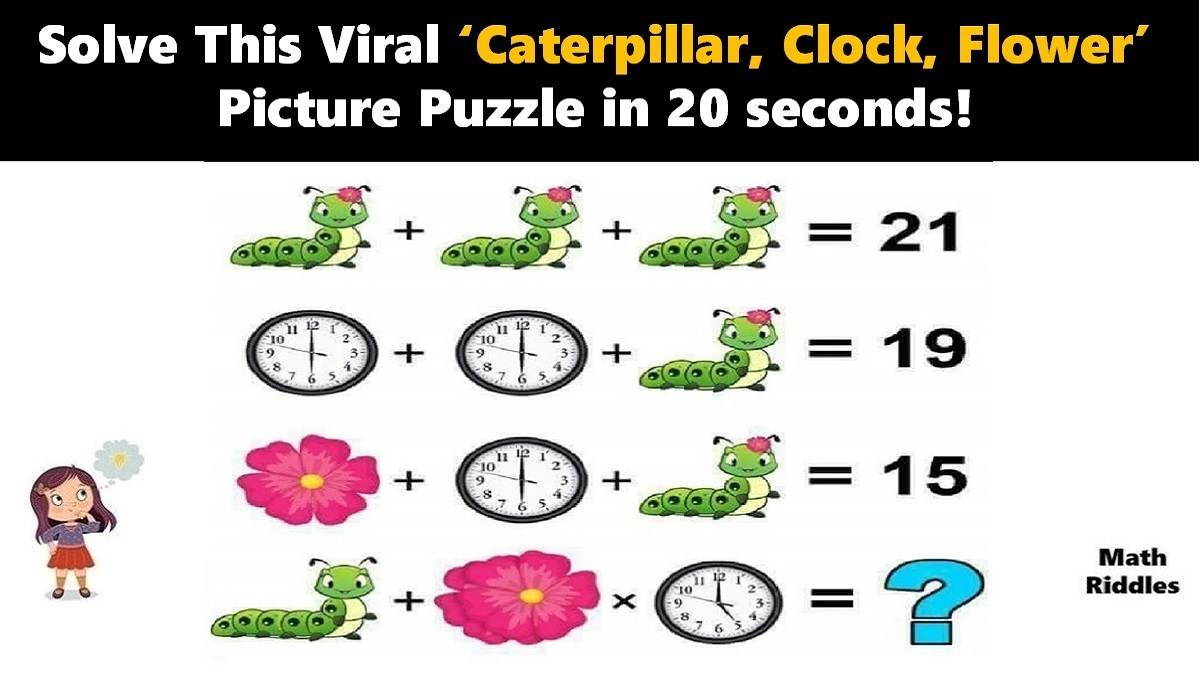# Math Riddles: Can You Solve This Viral ‘Caterpillar, Clock, Flower’ Picture Puzzle in 20 Seconds?

Math Picture Puzzles: Find out how brilliant you are. Only 1% of people with a high IQ can solve this tricky ‘Caterpillar, Clock, Flower’ math picture puzzle in 20 seconds.Math Riddles: Can You Solve This Viral ‘Caterpillar, Clock, Flower’ Picture Puzzle in 20 Seconds?

Math Riddles are so challenging, but that makes them worthwhile to solve. Math riddles are logical problems that require strong analytical abilities, high IQ, knowledge of math concepts, and good calculation skills. Math riddles have also been known to make Mathematics enjoyable for kids, students, and even adults to solve. Riddles, puzzles, and brain teasers can develop strategic thinking, logical reasoning, and problem-solving skills.

Today, we bring you another math picture puzzle that is all over social media. This ‘Caterpillar, Clock, Flower’ math picture puzzle has attracted more millions of answers but very few got it right.

Can you solve this ‘Caterpillar, Clock, Flower’ math picture puzzle? You have 20 seconds!

## Caterpillar, Clock, Flower Math Puzzle with Answers

Math Picture Puzzle #1

Row 1: There are three caterpillars (with 5 nodes and 1 flower) which equal to 21.

1 caterpillar + 1 caterpillar + 1 caterpillar = 21

=> 3 (caterpillars) = 21

=> 1 caterpillar = 21 / 3

=> 1 caterpillar (with 5 nodes and 1 flower) = 7

Now, we will use the value of 1 caterpillar (with 5 nodes and 1 flower) to solve the next rows of problems.

Row 2: Now, we have 1 clock (with 6’o clock) + 1 clock (with 6’o clock) + 1 caterpillar = 19. We know that 1 caterpillar (with 5 nodes and 1 flower) equals 7, so we apply that value accordingly.

1 clock (with 6’o clock) + 1 clock (with 6’o clock) + 1 caterpillar = 19

=> 2 (clock with 6’o clock) + 7 = 19

=> 2 (clock with 6’o clock) = 19 – 7

=> 2 (clock with 6’o clock) = 12

=> 1 clock with 6’o clock -= 12 / 2

=> 1 clock with 6’o clock = 6

Now, we will use the value of 1 clock (with 6’o clock) to solve the next rows of problems.

Row 3: There are 1 flower + 1 clock (with 6’o clock) + 1 caterpillar (with 5 nodes and 1 flower) = 15

We already know that value of 1 caterpillar (with 5 nodes and 1 flower) is 7 and the value of 1 clock (with 6’o clock) is 6. So, we apply the values accordingly.

1 flower + 1 clock (with 6’o clock) + 1 caterpillar (with 5 nodes and 1 flower) = 15

=> 1 flower + 6 + 7 = 15

=> 1 flower = 15 – 13

=> 1 flower = 2

Therefore, so far, we have solved that the value of 1 caterpillar (with 5 nodes and 1 flower) is 7, the value of 1 clock (with 6’o clock) is 6, and value of 1 flower is 2. Moving ahead, now we solve next row to figure out the final answer of this picture puzzle.

Row 4: Now, we reach the final question of the puzzle where we need to find out what the total of the final equation. Hence, we apply the values of each item that we have ascertained from the previous rows of this puzzle to find out the final sum.

Now, this is where 99% people fail to find the right answer. Notice, the caterpillar is with no flower and also there are 6 nodes instead of 5. Next, there are two flowers and the time in the clock is 5’o clock instead of 6’o clock. Let us put it together in an equation for simple understanding.

1 caterpillar (with 6 nodes and no flower) + 2 flowers x 1 clock (with 5’o clock) = ?

We will use the values that we have ascertained in the previous equations. Let us find the value of the 1 caterpillar (with 6 nodes and no flower). We know that the caterpillar (with 5 nodes and 1 flower) was 7 and the value of 1 flower was 2. So, first will deduct the value of 1 flower here. So, we get that 1 caterpillar (with 5 nodes) – 1 flower = 7 – 2 = 5. Accordingly, the value of 1 caterpillar (with 6 nodes and no flower) will be 6.

Next, the value of two flowers and 1 clock (with 5’o clock) time should be simple to find out. If value of 1 flower was 2 then the value of 2 flowers will be 4 and the value of 1 clock (with 6’o clock) time was 6 so the value of 1 clock (with 5’0 clock) time should be 5, right? Yes.

Now, let us put all the values and find the final answer.

1 caterpillar with 6 nodes and no flower + 2 flowers x 1 clock with 5’o clock = ?

=> 6 + 4 x 5 = ?

Apply BODMAS.

=> 6 + 20 = ?

=> 26